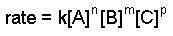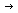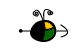Rate Laws and Order of a Reaction

Determining the Order of a Reaction from Its Rate Law

We need to know the rate law of a reaction in order to determine:

• The order of the reaction with respect to one or more reactants.
• The overall order of the reaction.

For the rate law• Order with respect to A = n
• Order with respect to B = m
• Order with respect to C = p
• Reaction order, or overall order = n + m + p

Note: the stoichiometric coefficient in the balanced equation for a chemical reaction is usually different from the order.

reactionexperimental rate laworder
NO2 + CONO + CO2 rate = k[NO2]2 2nd order reaction
2nd order in NO2
0 order in CO
CH3CHOCH4 + CO rate = k[CH3CHO]2 2nd order reaction
2nd order in CH3CHO

Top